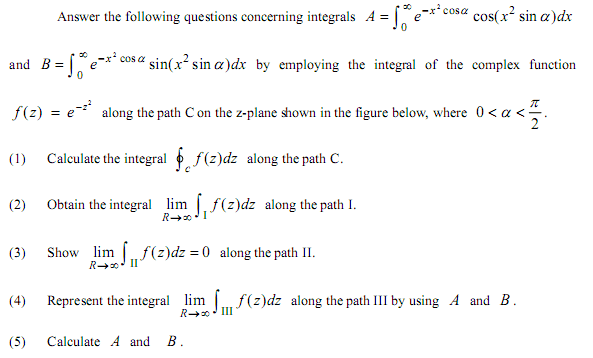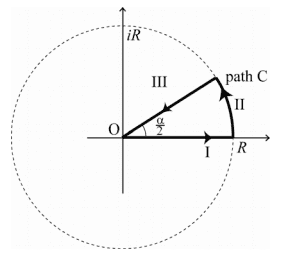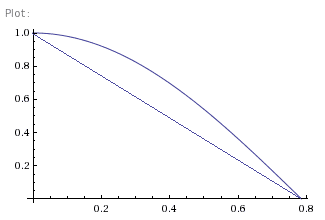# Contour integrals: Problem

• degs2k4

## Homework Statement## The Attempt at a Solution

ap1)

0, since there are no singularities for the function inside contour

ap2)

Provided that INT{-infinite,infinite} e^-z^2dz = sqrt(pi) -gaussian integral-,
INT{0,infinite} e^-z^2dz = sqrt(pi)/2

ap3)

show that int(arc)-> 0 as R->infinite. Using parametrization of z = R*e^(i*B) where 0<=B<=alpha/2, dz = i* R*e^(i*B) dB we can substitute z and dz :

INT{0,alpha/2} e^-(R*e^(i*B))^2 * i*R*e^(i*B) dB =
= INT{0,alpha/2} i*R*e^(i*B) / e^(R^2 * e^(2*i*B)) dB
hence, we have arrived to something of the form R / e^(R^2),
and if R->infinite, the integral -> zero

(Note: I think that this may not be valid because we need an ML inequality
to demonstrate this. However I don't know how to do this in this problem )

ap4)

Using parametrization: z = r*e^(i*alpa/2) with r from R to 0,
dz = e^(i*alpha/2) dr,
and e^(ai) = cos a + i * sin a,
I want to transform INT(III) 1/e^z^2 dz so that we can have integrals A and B inside.
Is this way of proceeding correct? I have tried transforming the integral but haven't been
able to get to A and/or B.

Any kind of help or directions will be greatly appreciated.

Use absolute values for III:

$\left|\int_0^{\theta} iRe^{(-R^2e^{it})^2}e^{it}dt\right|\leq \int_0^{\theta}\left|Re^{-R^2\cos(2t)}\right|dt$

Now, draw a picture of cos(2t) from 0 to pi/4. Next draw a straight line from the point (0,1) to (pi/4,0). Now in that region cos(2t)>myline which means:

$e^{-R^2\cos(2t)} <e^{-R^2(myline)}$

but you can integrate the myline version easily and take the limit as R goes to infinity of that one. What happens when you do that?

Last edited:

I guess you are referring to ap 3), with contour integral in II, using the parametrization:

$$z=Re^{it}, where 0 \leq t \leq \theta$$
$$dz=iRe^{it} db$$

doing so, we get:

$$\int_0^{\theta} e^{(-R^2e^{2it})}iRe^{it}dt$$

which I think is different (?) from what you write...Anyway, plotting the line and curve you requested:I understand that cos 2t > "myline", and therefore

$$e^{-R^2\cos(2t)} \leq e^{-R^2\cos(myline)}$$

I guess that when R tends to infinite, 1/e^R tends to 0 and thus the integral tends to 0...

That's the same as mine isn't it? Now just follow through with the integral in myline:

\begin{aligned} \left|\int_0^{\theta} iRe^{(-R^2e^{it})^2}e^{it}dt\right|& \leq \int_0^{\theta}\left|Re^{-R^2\cos(2t)}\right|dt\\ &\leq \int_0^{\theta}R e^{-R^2(-4/\pi(x-\pi/4))}dx \\ \end{aligned}
and that last one is easy to integrate and then you can take it's limit as R goes to infinity.

In the first part of the left, you wrote this:

$$\int_0^{\theta} iRe^{(-R^2e^{it})^2}e^{it}dt$$

and I wrote this:

$$\int_0^{\theta} e^{(-R^2e^{2it})}iRe^{it}dt$$

which I think is different (you have the -R^2 powered by 2 and thus becoming R^4) ?

Anyway, I understand your way of solving this but I'm sure I wouldn't ever tried to solve it this way. Was my first attempt of the first post incorrect ? Why?

Thanks again!

In the first part of the left, you wrote this:

$$\int_0^{\theta} iRe^{(-R^2e^{it})^2}e^{it}dt$$

and I wrote this:

$$\int_0^{\theta} e^{(-R^2e^{2it})}iRe^{it}dt$$

which I think is different (you have the -R^2 powered by 2 and thus becoming R^4) ?

Was my first attempt of the first post incorrect ? Why?

Thanks again!

In general I think you were on the right track but you were not specific enough although it's was hard for me to interpret your work. Need to show precisely why it's going to zero and I wouldn't have thought of that either except I have Schaum's Complex Variables which go over a similar problem which I then just applied to this one. Try and get Schaum's. I find it useful.

Thanks! I'll look into it and try again the following parts of the problem (4,5). Anyway, still stuck in 4...If I get any further advance in this problem I'll post again in this thread...

Just split up the integral into its real and imaginary part along III:

$\mathop\int\limits_{z=re^{it}} e^{-z^2}dz=e^{it}\int_{\infty}^0 e^{-r^2(\cos(2t)+i\sin(2t))}dr$

where t is in the desired range or just adjust the angle parameters to fit the reqirements. This is all based on the fact that sin and cos are positive in (0,pi/2).

Last edited:
Thanks again, Jackmell.

I tried what you said and here are the results:

$$\int\limits_{z=re^{it}} e^{-z^2}dz=e^{it}\int_{R}^0 e^{-r^2(\cos(2t)+i\sin(2t))}dr=e^{it}\int_{R}^0 e^{-r^2(\cos(2t)} e^{-r^2 i(\sin(2t))}dr=$$

$$= e^{it}\int_{R}^0 e^{-r^2(\cos(2t)} cos(-r^2(\sin 2t)) + i\sin(-r^2(\sin 2t)) dr =-e^{it}\int_{0}^R \frac{cos(-r^2(\sin 2t))}{e^{r^2(\cos(2t)}} dr - e^{it}\int_{0}^R \frac{i\sin(-r^2(\sin 2t))}{e^{r^2(\cos(2t)}} dr$$

and taking the real part and when R-> infinite:

$$-e^{t}\int_{0}^\infty \frac{cos(-r^2(\sin 2t))}{e^{r^2(\cos(2t)}} dr - e^{t}\int_{0}^\infty \frac{sin(-r^2(\sin 2t))}{e^{r^2(\cos(2t)}} dr = -e^{t} A - e^{t} B$$

which seems to be the sol for ap (4).

Now, when it comes to ap (5), A and B calculations, we can say that:

$$0 = \frac{\sqrt\pi}{2} + 0 - cos(t)A - cos(t)B = \frac{\sqrt\pi}{2} - cos(\frac{\alpha}{2})A - cos(\frac{\alpha}{2} )B$$

However, I can't see again, how to go any further...

Firstly, cos(-poo)=cos(poo).
sin(-poo)=-sin(poo)
Try and tidy up your signs.

Don't take real parts yet (I think you did that wrong anyway - I'm not sure what you did).
When you do take real and imaginary parts, you might have to write e^it as (c+is) and multiply it out to check for cross terms, if you want to do that (though there's a slightly shorter way if you delay doing so).

You're aiming to get something like (signs and constants are invariably incorrect, just giving an example form of a step)
$$e^{i\alpha} (A-iB)=\sqrt{\pi}$$
(and could you see how to get A and B from that?)

--
Oh, I guess I'll mark what you did in that last post.

$$\int\limits_{z=re^{it}} e^{-z^2}dz = ...=-e^{it}\int_{0}^R \frac{cos(-r^2(\sin 2t))}{e^{r^2(\cos(2t)}} dr - e^{it}\int_{0}^R \frac{i\sin(-r^2(\sin 2t))}{e^{r^2(\cos(2t)}} dr$$
seems fine. LHS is what you want to represent. RHS is something in terms of A and B (and some complex constants).

Last edited:
Jerbearrrrrr:

Thanks for your reply. So redoing again the last steps of the previous post:

$$-e^{it}\int_{0}^\infty \frac{cos(-r^2(\sin 2t))}{e^{r^2(\cos(2t)}} dr - i e^{it}\int_{0}^\infty \frac{sin(-r^2(\sin 2t))}{e^{r^2(\cos(2t)}} dr = -e^{it} ( A + i B )$$

and then :

$$\frac{\sqrt\pi}{2} = e^{it} ( A + i B )$$

But here I don't know what to do...I guess some property of complex numbers that I can't remember can be applied ?

Last edited:
Thanks again, Jackmell.

I tried what you said and here are the results:

$$\int\limits_{z=re^{it}} e^{-z^2}dz=e^{it}\int_{R}^0 e^{-r^2(\cos(2t)+i\sin(2t))}dr=e^{it}\int_{R}^0 e^{-r^2(\cos(2t)} e^{-r^2 i(\sin(2t))}dr=$$

$$= e^{it}\int_{R}^0 e^{-r^2(\cos(2t)} cos(-r^2(\sin 2t)) + i\sin(-r^2(\sin 2t)) dr =-e^{it}\int_{0}^R \frac{cos(-r^2(\sin 2t))}{e^{r^2(\cos(2t)}} dr - e^{it}\int_{0}^R \frac{i\sin(-r^2(\sin 2t))}{e^{r^2(\cos(2t)}} dr$$

and taking the real part and when R-> infinite:

$$-e^{t}\int_{0}^\infty \frac{cos(-r^2(\sin 2t))}{e^{r^2(\cos(2t)}} dr - e^{t}\int_{0}^\infty \frac{sin(-r^2(\sin 2t))}{e^{r^2(\cos(2t)}} dr = -e^{t} A - e^{t} B$$

which seems to be the sol for ap (4).

Now, when it comes to ap (5), A and B calculations, we can say that:

$$0 = \frac{\sqrt\pi}{2} + 0 - cos(t)A - cos(t)B = \frac{\sqrt\pi}{2} - cos(\frac{\alpha}{2})A - cos(\frac{\alpha}{2} )B$$

However, I can't see again, how to go any further...

I get for III:

$$\mathop\int\limits_{III} e^{-z^2}dz=e^{it}\left\{\int_{\infty}^0 e^{-r^2\cos(2t)}\cos(r^2\sin(2t))dr-i\int_{\infty}^0 e^{-r^2\cos(2t)}\sin(r^2\sin(2t))dr\right\}$$

$$\mathop\int\limits_{III} e^{-z^2}dz==-e^{it}(A-iB)$$

combine all the contours to get:

$$\frac{\sqrt{\pi}}{2}=e^{it}(A-iB)=(\sigma+i\gamma)(A-iB)$$

That means the imaginary part on the right side is zero and the real part is $$\frac{\sqrt{\pi}}{2}$$

What then does that say about A and B, i.e., can you figure out what A and B are from what that said?

Last edited:
It's a better idea to jiggery pokery the e^it onto the other side.
Then as A and B are real (why?), you can simply read them off from the LHS!
$$\frac{\sqrt{\pi}}{2} (\cos t - i\sin t) =A+iB$$
(sign errors in my working - the signs are left as an exercise for you)

I think that the correct signs are like this:

$$\frac{\sqrt{\pi}}{2}=e^{it}(A-iB)$$

I guess that:

$$\frac{\sqrt{\pi}}{2}e^{-it} =\frac{\sqrt{\pi}}{2}\cos{t} - i\frac{\sqrt{\pi}}{2}\sin{t}=(A-iB)$$

And thus:

$$A=\frac{\sqrt{\pi}}{2}\cos{t}$$

$$B=\frac{\sqrt{\pi}}{2}\sin{t}$$

maybe?

Last edited:
That's correct but you should also try to learn how to check it yourself either numerically or exactly using any of the CAS tools like Matlab, Mathematica, etc. That would give you some additional confidence the analytic answer is correct.

Thanks!

Thanks for the advice. It is the first time I am studying complex numbers with those contour integrals, moreover I am not used to CAS software...yet

Thanks for everything jackmell & jerbearrrrrr! Your help was priceless!